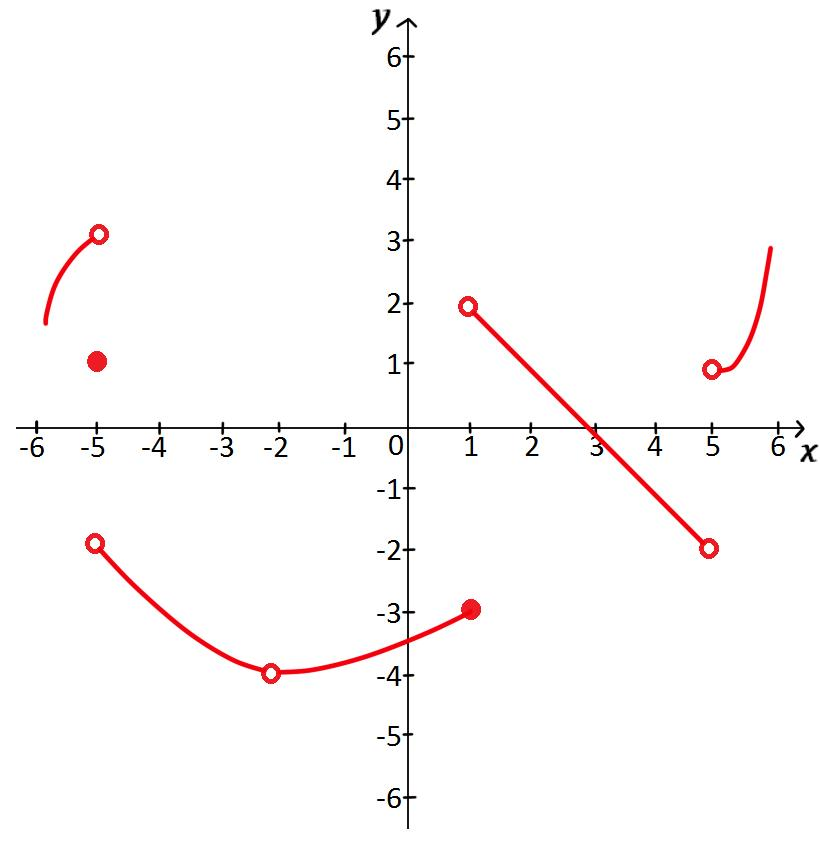# Finding limits from graphs##### Examples
###### Lessons
1. For the function f whose graph is shown, state the following:1. $\lim_{x \to -5^-} f(x)$
$\lim_{x \to -5^+} f(x)$
$\lim_{x \to -5} f(x)$
$f(-5)$
2. $\lim_{x \to -2^-} f(x)$
$\lim_{x \to -2^+} f(x)$
$\lim_{x \to -2} f(x)$
$f(-2)$
3. $\lim_{x \to 1^-} f(x)$
$\lim_{x \to 1^+} f(x)$
$\lim_{x \to 1} f(x)$
$f(1)$
4. $\lim_{x \to 4^-} f(x)$
$\lim_{x \to 4^+} f(x)$
$\lim_{x \to 4} f(x)$
$f(4)$
5. $\lim_{x \to 5^-} f(x)$
$\lim_{x \to 5^+} f(x)$
$\lim_{x \to 5} f(x)$
$f(5)$
###### Free to Join!
StudyPug is a learning help platform covering math and science from grade 4 all the way to second year university. Our video tutorials, unlimited practice problems, and step-by-step explanations provide you or your child with all the help you need to master concepts. On top of that, it's fun - with achievements, customizable avatars, and awards to keep you motivated.
• #### Easily See Your ProgressWe track the progress you've made on a topic so you know what you've done. From the course view you can easily see what topics have what and the progress you've made on them. Fill the rings to completely master that section or mouse over the icon to see more details.
• #### Make Use of Our Learning Aids###### Practice Accuracy

See how well your practice sessions are going over time.

Stay on track with our daily recommendations.

• #### Earn Achievements as You LearnMake the most of your time as you use StudyPug to help you achieve your goals. Earn fun little badges the more you watch, practice, and use our service.
• #### Create and Customize Your AvatarPlay with our fun little avatar builder to create and customize your own avatar on StudyPug. Choose your face, eye colour, hair colour and style, and background. Unlock more options the more you use StudyPug.
###### Topic Notes
Limit is an important instrument that helps us understand ideas in the realm of Calculus. In this section, we will learn how to find the limit of a function graphically using one-sided limits and two-sided limits.
DEFINITION:
left-hand limit: $\lim_{x \to a^-} f(x) = L$
We say "the limit of f(x), as x approaches a from the negative direction, equals L".
It means that the value of f(x) becomes closer and closer to L as x approaches a from the left, but x is not equal to a.

DEFINITION:
right-hand limit: $\lim_{x \to a^+} f(x) = L$
We say "the limit of f(x), as x approaches a from the positive direction, equals L".
It means that the value of f(x) becomes closer and closer to L as x approaches a from the right, but x is not equal to a.

DEFINITION:
$\lim_{x \to a} f(x) = L$ if and only if $\lim_{x \to a^+} f(x) = L$ and $\lim_{x \to a^-} f(x) = L$